# N-ary Tree Data Structure

We know that a binary tree is a rooted tree in which each node has no more than two children. We can extend this definition to an n-ary tree. If a tree is rooted in which each node has no more than N children, it is called an n-ary tree. In other words, n-ary trees are tree data structures with up to n children nodes for each node.

The following image shows an example of a 3-ary tree: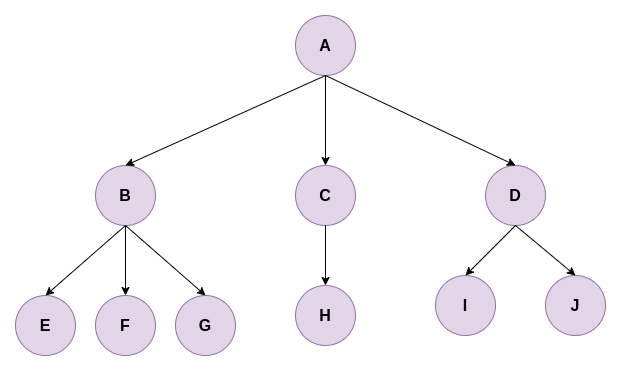### How to represent an n-ary tree?

For each node of the n-ary tree, we need two pieces of information:

1. Value of the node
2. Address of its child nodes

We can use an Array or LinkedList to store the information about a node’s children. However, there are some drawbacks to both of these methods. If we decide to store information about children of a node in an array, we can only store a fixed number of children’s addresses as we may not know the number of children of a node. Also, if we decide to keep an array of lengths equal to the maximum number of children of a node, it might lead to wastage of space. For example, some nodes have only one or two children, whereas others have n(>5) children. If we use an array of length n in all nodes, the nodes with lesser children will not need an array of length n to store information about their children’s addresses.

To avoid this wastage of space, let’s say we decide to use a LinkedList. Now we can dynamically add nodes whenever required, and we do not need to preallocate space for storing information about children in a node. Nevertheless, this method too has a draw drawback. Try to think about it. What will happen if we want to access a child of a node? It will take O(n) time! As the number of children(n) increases, the time to access children from a node will increase linearly. We tried to optimize the space required, but the time complexity increased.

We will use dynamic arrays(vector in C++, ArrayList in Java, list in Python) to store information about children in a node to optimize time and space complexities. With this approach, we can access any child’s address in O(1) and do not have to know the number of children of each node beforehand.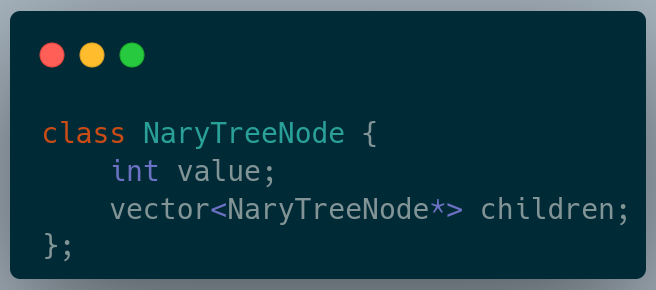### The optimal approach for N-ary tree representation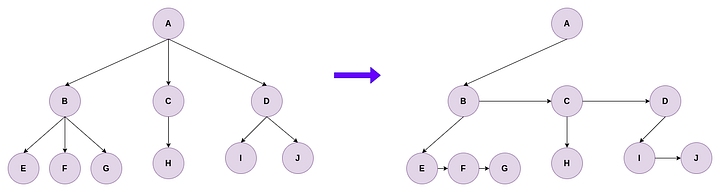As shown in the above image, we can try to convert an n-ary tree into a binary tree by removing links from a parent node to each child node. We can keep only two links for each node: a link to the first child and the next sibling node. Sibling nodes are nodes at a level having the same parent. We can convert an n-ary tree to this representation with the following steps:

1. For each node, link the sibling nodes(children of the same parent) from left to right.
2. Remove links from parent to all children, except the node’s first child.

We can see that as no extra links are required, this method is space-efficient. Also, all the nodes are of constant size, and we do not need dynamic arrays to store information about children. With the above representation, all n-ary nodes can be seen as binary trees, which are easy to visualize and operate on.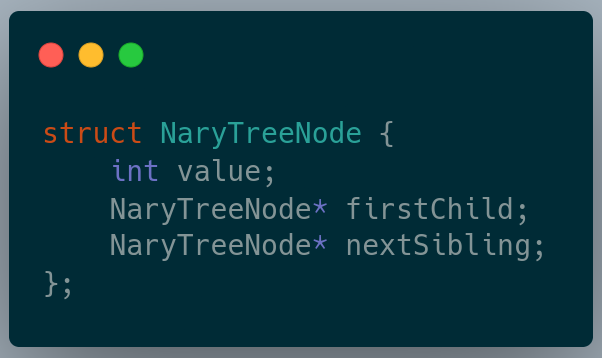This method is discussed in detail below. We will discuss all the operations on an n-ary tree considering the second representation of a node(using dynamic arrays). Before looking at the different operations of an n-ary tree, let’s look at the different types of n-ary trees.

### Types of n-ary trees

#### Full n-ary tree

A full n-ary tree is an n-ary tree that allows each node to have either 0 or n children. The following image shows a pictorial representation of a full n-ary tree.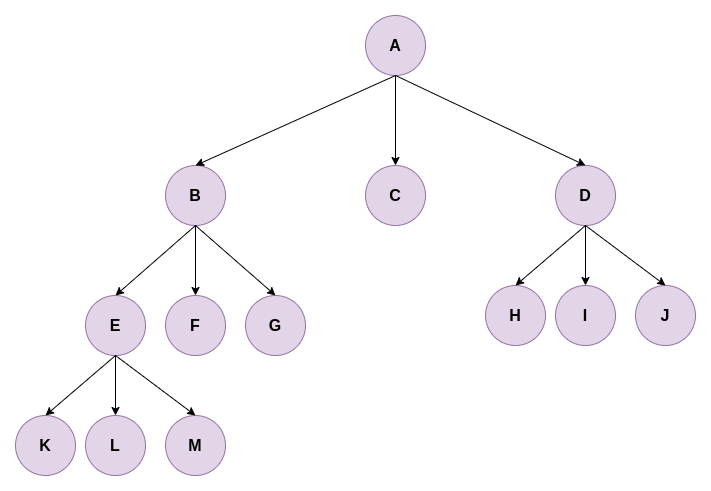In the above image, all nodes have either 0 or 3 children.

#### Complete n-ary tree

A complete n-ary tree is an n-ary tree in which the nodes at each tree level should have exactly n children(they are complete), except for the nodes at the last level. If the nodes at the last level are not complete, the nodes must be as left as possible. The following image shows a pictorial representation of a complete n-ary tree.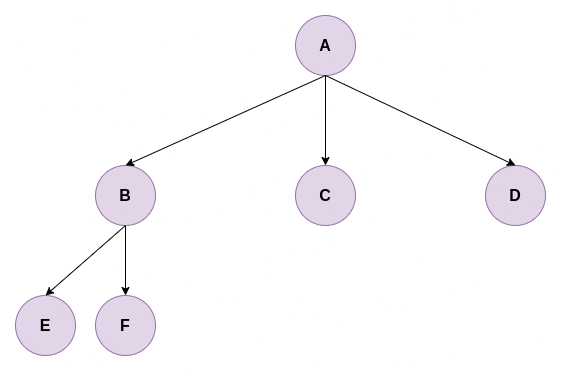In the above image, all nodes(except for the last level) have three children, and the incomplete nodes are as left as possible.

#### Perfect n-ary tree

A perfect n-ary tree is a full n-ary tree, but the leaf nodes’ levels must be the same. The following image shows a pictorial representation of a perfect n-ary tree.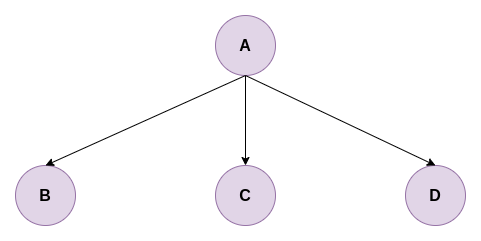The following n-ary tree will be considered for discussing examples for different operations on the n-ary tree.### Preorder traversal of n-ary tree

In a preorder traversal of an n-ary tree, the root node is visited; after that, the subtrees are traversed recursively from left to right. As the root node is traversed before (or pre) any subtree, it is called preorder traversal.

Example output : [A, B, E, F, C, D]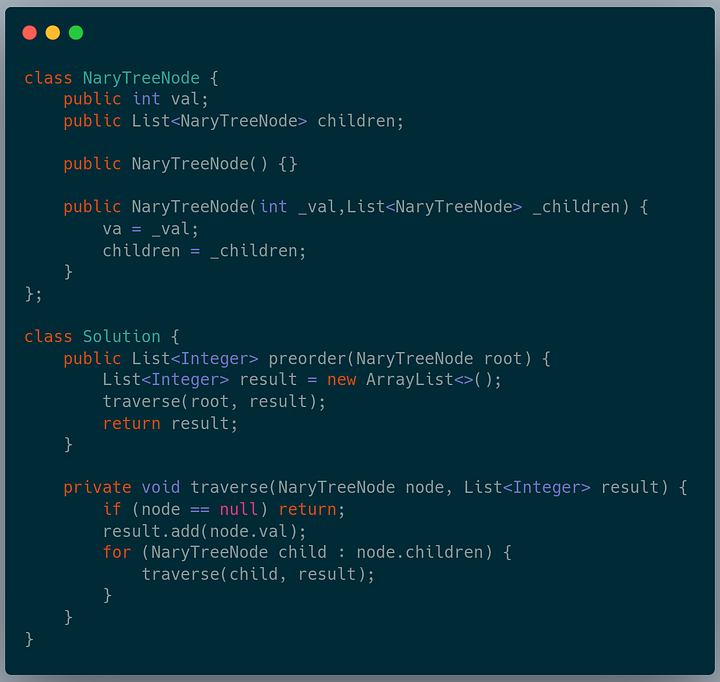### Postorder traversal of n-ary tree

Postorder traversal is a traversal in which we first traverse the subtrees recursively in a postorder traversal and visit the root node at the end.

Example output : [E, F, B, C, D, A]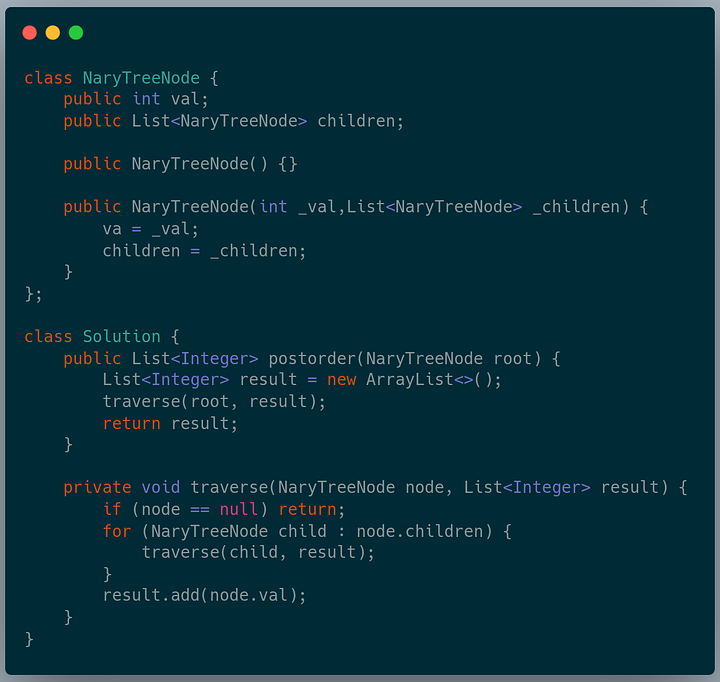### Level order traversal of n-ary tree

In a level order traversal, we process all tree nodes by depth: first the root, then the children of the root, and so on. This process is equivalent to a breadth-first search from the root.

Example output: [[A], [B, C, D], [E, F]]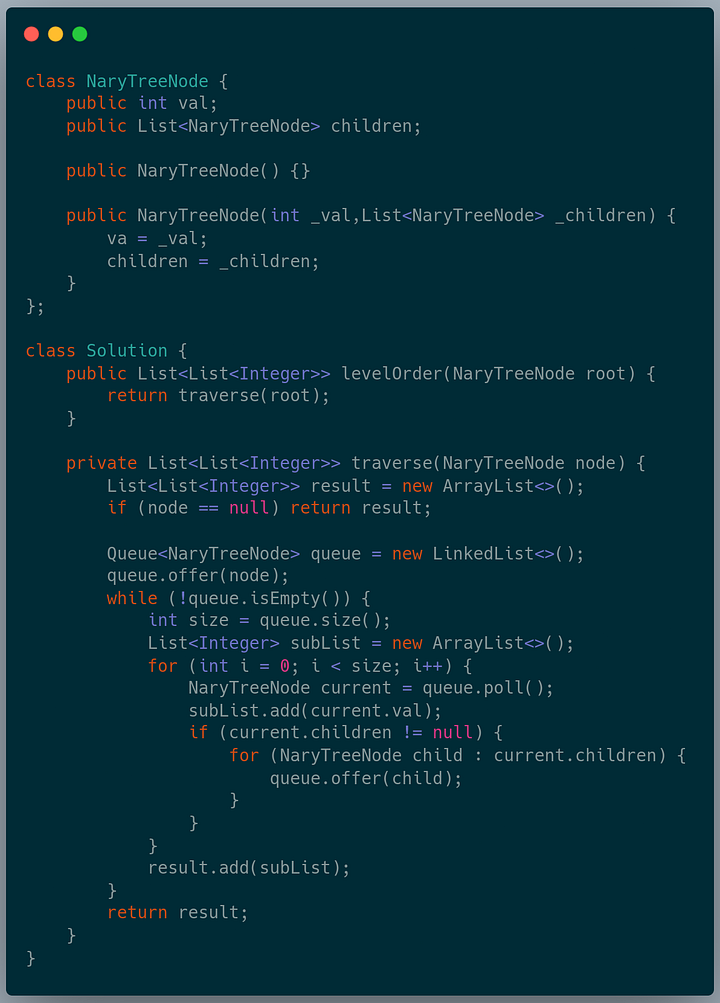### Maximum depth of the n-ary tree

The maximum depth of an n-ary tree is also called the height of the n-ary tree. The maximum depth is the number of nodes along the longest path from the root node down to the farthest leaf node.

Example output : 3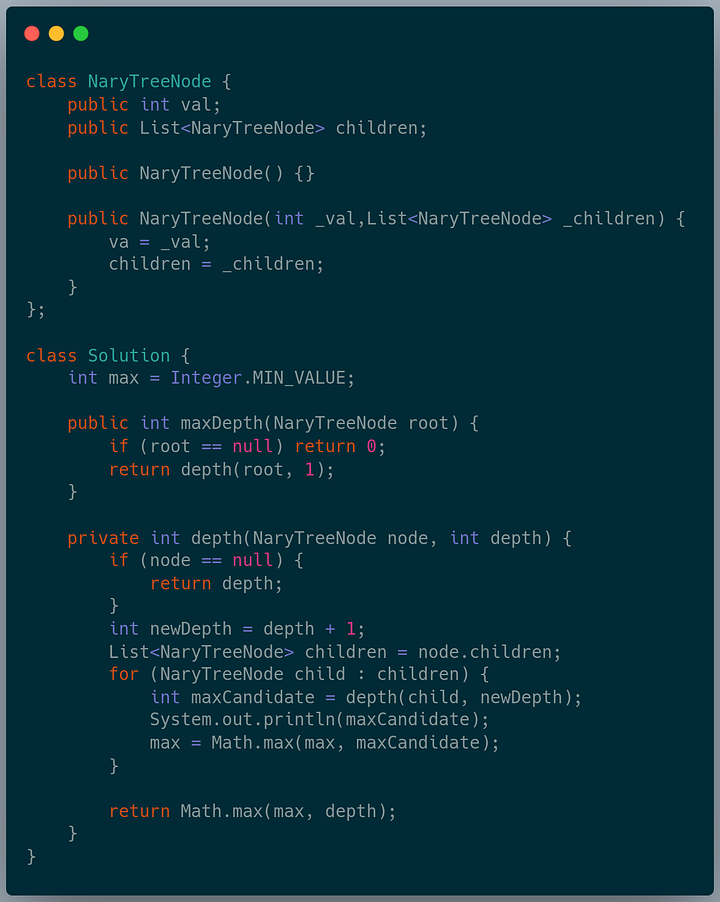### Convert an n-ary tree to a binary tree

We have seen that storing only two things, the first child and next sibling of a node in an n-ary tree, results in the most space-efficient way of representing an n-ary tree node. We are converting the n-ary tree into a binary one using this representation.

Converting an arbitrary n-ary tree to a binary tree would only increase the tree’s height. Let us see by how much the height increase. Suppose we have a perfect n-ary tree with m nodes. We know that in a perfect n-ary tree, all nodes have 0 or n children, with the last level being the same. Let the height of the tree be h. The following condition holds for a perfect n-ary tree with m nodes of height h.This simplifies to:We get the following result, where D is the depth of the n-ary tree.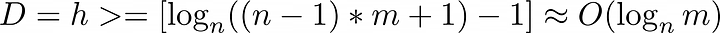Now, if we convert the n-ary tree to a binary tree, the number of nodes, m, will remain the same. The depth, in that case, will be O(log2 m).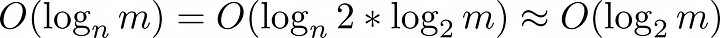From the above calculations, we can conclude that the tree height increases by a constant factor and would not affect the worst-case time complexity.

To convert an n-ary tree to a binary tree, first, we will link all the immediate children nodes of a given parent node together to form a linked list. Then, we keep the link from the parent to the first child(the leftmost child) and remove all the other links to the rest of the children. Repeat this process for all the children until we have processed all the internal nodes and rotate the tree 45 degrees clockwise. The tree obtained is the desired binary tree representation of the given n-ary tree.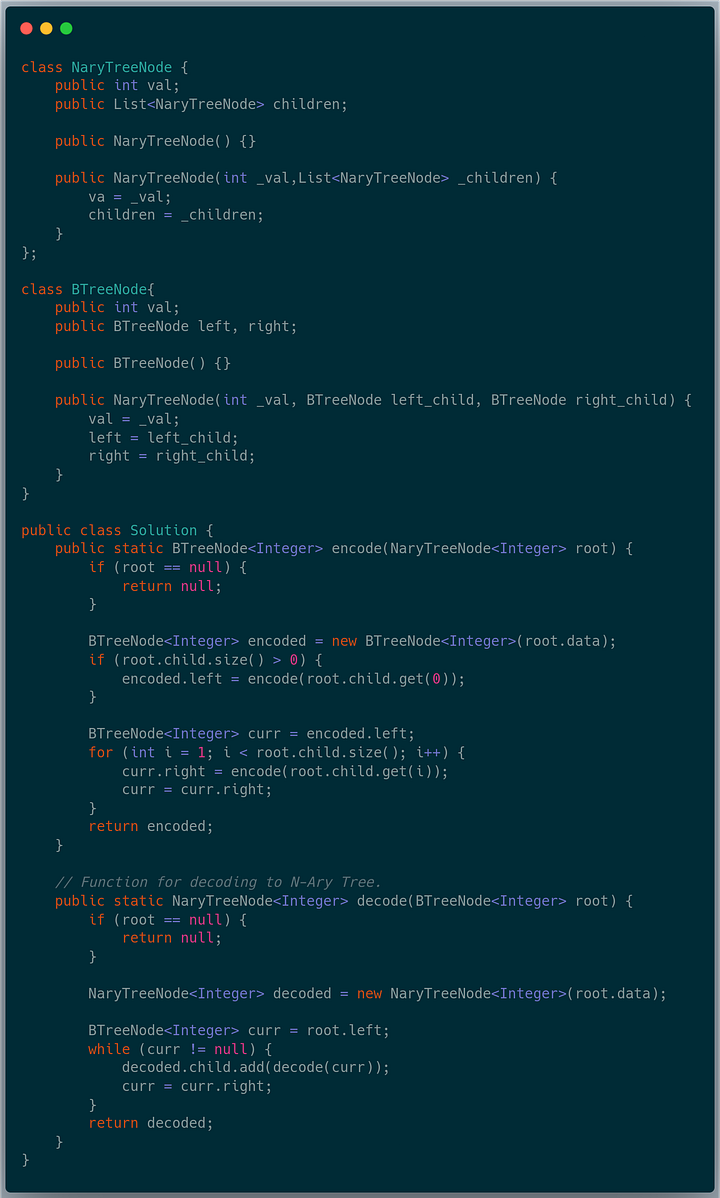Enjoy learning, Enjoy Algorithms!

Share on social media:

© 2022 Code Algorithms Pvt. Ltd.